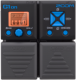Discussion in 'Zoom G1on/G1Xon' started by Tonelib, May 20, 2018.Device: Zoom G1on
Firmware: 1.21

Optimized for: Phones/Speaker

Effects chain:This re-creates a rich lead tone that is indispensable to the LA sound.

Effect: "Booster" (Overdrive / Distortion), active - "yes"
"Gain" = 83
"Tone" = 26
"Level" = 103

Effect: "BGN DRIVE" (Amp simulators), active - "yes"
"Gain" = 73
"Tube" = 15
"Level" = 100
"Trebl" = 41
"Middl" = 54
"Bass" = 40
"Prese" = 62
"CAB" = DZ DRIVE 4x12
"OUT" = LINE

Effect: "ParaEQ" (Dynamics / Filter), active - "yes"
"Freq1" = 6.3kHz
"Q1" = 2
"Gain1" = 4
"Freq2" = 2.0kHz
"Q2" = 8
"Gain2" = -8
"Level" = 100

Effect: "Delay" (Delay / Reverb), active - "yes"
"Time" = 560
"F.B" = 37
"Mix" = 40
"HiDMP" = 5
"P-P" = MONO
"Level" = 100
"Tail" = On

Effect: "Hall" (Delay / Reverb), active - "yes"
"Decay" = 18
"Tone" = 10
"Mix" = 38
"PreD" = 16
"Level" = 100
"Tail" = Off

Patch Volume: 90

Note: This is a patch file, you will need to download and install the ToneLib software to use the patch.